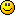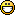Topic: How to make buttons in Maratis

Hi, everyone

I know that there are some discussions about how to make buttons in Maratis. Unfortunately, after doing some search and test, I still can't create buttons using rayHit (sorry for my noobiness). So I tried to follow this tutorial about getUnProjectedPoint but I got stuck again.
In my scene, I have three buttons: moveLeft, rotate, and moveRight. I want to use those buttons to move and rotate a cube.

Following the tutorial, I can make the rotation button to rotate the cube. However, when I moved the mouse cursor over the other buttons (moveLeft and moveRight), the cube also rotating. Can someone give me a hint of how to fix my script?

And here is the script based on the documentation:

moveRight = getObject("moveRight")
moveLeft = getObject("moveLeft")
Rotate = getObject("Rotate")
Cube = getObject("Cube")
Camera = getObject("Camera")

point = getUnProjectedPoint(Camera, vec3(x, y, z))

function onSceneUpdate()
mx = getAxis("MOUSE_X")
my = getAxis("MOUSE_Y")

V1 = getUnProjectedPoint(Camera, vec3(mx, my, 0))
V2 = getUnProjectedPoint(Camera, vec3(mx, my, 1))

point, Rotate = rayHit(V1, V2)

if point then
rotate(Cube, {0, 0, 1}, 4)
end

--[[
if point then
translate(Cube, {1, 0, 0}, 2)
end
]]--

end

Thanks for any help.

Re: How to make buttons in Maratis

Hey Xone!

You need to move

point = getUnProjectedPoint(Camera, vec3(x, y, z))

into your "onSceneUpdate" function so it updates every frame with the new cursor position.
Then you still have to check which button you have clicked on:

point, object = rayHit(V1, V2)
if point then
if object == Rotate then
-- Do rotation
elseif object == moveLeft then
-- Move left
elseif object == moveRight then
-- Move right
end
end

This should do it.
If you have further question then just ask them. You don't need to be afraid because you think you are a "noob"Hope this helpsSponk

Re: How to make buttons in Maratis

Ah, yes, it's working!
Many, many thanks, Sponk! That helps a lot.
It's solved now. Thank you.

Re: How to make buttons in Maratis

Nice to hear